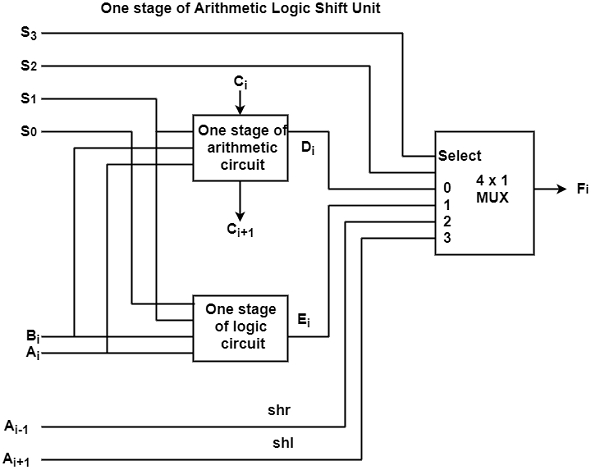# What is Arithmetic Logic Shift Unit in Computer Architecture?

A computer systems create use of several storage registers that are linked to a typical operational unit is known as the arithmetic and logic unit (ALU). ALU is the central and one of the most essential units internal the CPU of the computer.

All the logical and numerical operations of a computer are implemented here. The contents of a particular register are arranged in the input of ALU. ALU implements the given operation and then transfers it to the target register.

The one stage of an arithmetic logic shift unit is demonstrated in the figure. The subscript i nominate a frequent stage. There are two inputs are used for both the arithmetic and logic units.A specific micro-operation is choosing with inputs S1 and S0 A 4 x 1 multiplexer at the output select between an arithmetic output inand a logic output in . The data in the multiplexer are chosen with inputs S3 and S2.

There are two data inputs to the multiplexer receive inputs Ai-1 for the shift-right operation and Ai+1 for the shift-left operation. The circuit should be continued n times for an n-bit ALU.

The output carry Ci+1 of a given arithmetic stage should be linked to the input carry Ci of the next stage in the series. The input carries to the first stage is the input carry Cin It can support a selection variable for the arithmetic operations.

The circuit whose one stage is determined in the diagram. It provides eight arithmetic operations, four logic operations, and two-shift operations. Each operation is selected with the five variables S3,S2,S1,S0The input carries Ci∧Cin can be used for selecting an arithmetic operation only.

Functional Table for Arithmetic Logic Shift Unit

S3S2S1S0CinOperationFunction
00000F = ATransfer A
00001F = A + 1Increment A
00011F = A + B + 1Add with carry
00100F = A + B’Subtract with borrow
00101F = A + B’ + 1Subtraction
00110F = A – 1Decrement A
00111F = ATransfer A
0100XF = A $\wedge$ BAND
0101XF = A $\vee$ BOR
0110XF = A$\oplus$BXOR
0111XF = A’Complement A
10XXXF = shr AShift right A into F
11XXXF = shl AShift Left A into F

This table shows the 14 operations of the ALU. The first eight are arithmetic operations and are selected with S3S2 = 00. The next four are logic operations and are selected with S3S2 = 01. The final two operations are shift operations and are selected with S3S2 = 10 and 11. The other three selection inputs do no influence the shift.